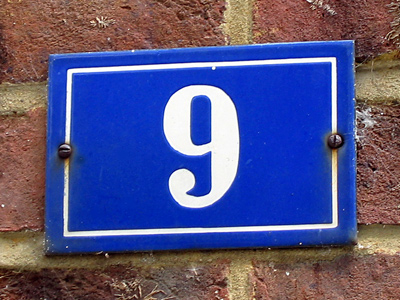A good strategy for adding 9 is to add 10 and take away 1.

# Addition and Subtraction (Year 3)

In Year 3, which is the first year in KS2 Maths, you'll learn cool tricks for adding and taking away numbers in Math. It'll make you super confident when you see plus or minus symbols.

Addition is when you put numbers together, like 10 girls + 7 boys = 17 friends at your party! And if you have 20 prizes, you'll have 3 extra because 20 - 17 = 3. See, you just did an addition and subtraction problem! It's helpful in real life too.

1.
75 subtract 40.
30
35
40
45
7 - 4 = 3 so 70 - 40 = 30
30 + 5 = 35
2.
Which is a good strategy for adding 9?
Add 20 and take away 11
Add 10 and take away 1
e.g. 35 + 9 so 35 + 10 = 45 then 45 - 1 = 44
3.
17
20
21
25
A good strategy would be 8 + 10 - 1 = 17
17 + 3 = 20 plus 1 left over = 21
4.
What is 78 + 56?
134
138
124
128
It might help to add the tens first and then the ones
5.
17 take away 8.
9
10
11
12
17 - 10 = 7
7 + 2 = 9
6.
Which is the missing number: 7 + __ = 20?
10
12
13
14
One way to work this out is to take 7 away from 20 which will give you the answer 13
7.
Which is the best method of calculating 45 + 46?
Add the tens first and then the ones
Count on from 45
Add the ones first and then the tens
45 x 2 = 90
90 + 1 = 91
8.
What is the sum of 14 and 8?
18
20
21
22
14 + 10 = 24
24 - 2 = 22
9.
Which number sentence is incorrect?
8 + 5 = 13
13 - 5 = 8
5 + 8 = 13
8 - 5 = 13
The other number sentence that would be correct is 13 - 8 = 5
10.
Which will not total 100?
65 + 35
55 + 45
75 + 35
85 + 15
75 + 35 = 110